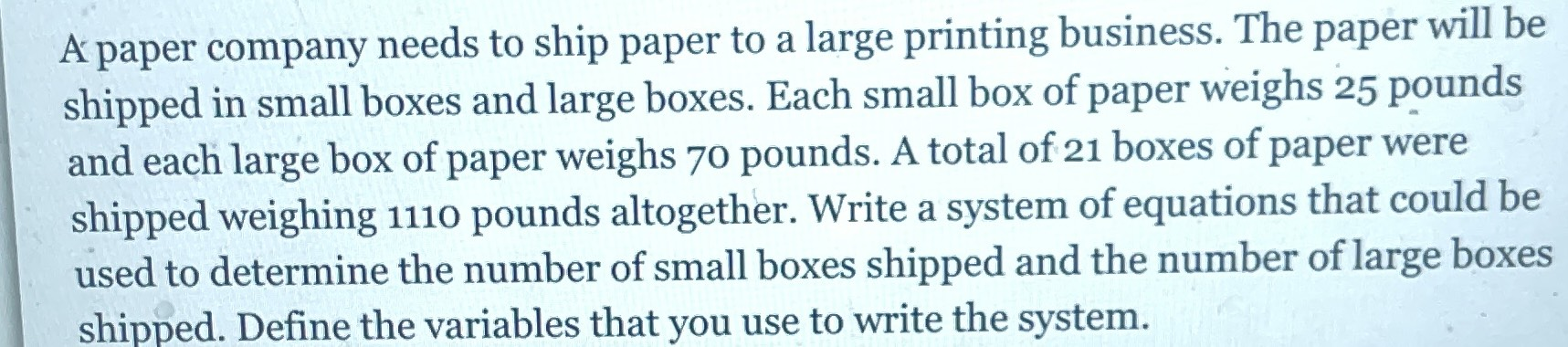### ¿Todavía tienes preguntas de matemáticas?

Pregunte a nuestros tutores expertos
Algebra
PreguntaA paper company needs to ship paper to a large printing business. The paper will be shipped in small boxes and large boxes. Each small box of paper weighs $$25$$ pounds and each large box of paper weighs $$70$$ pounds. A total of $$21$$ boxes of paper were shipped weighing $$1110$$ pounds altogether. Write a system of equations that could be used to determine the number of small boxes shipped and the number of large boxes shipped. Define the variables that you use to write the system.

x-----number of small boxes shipped

y------number of large boxes shipped

$$x + y = 21,25 x + 70 y = 1110 : x = 8 , y = 13$$

Steps

$$\left[ \begin{array} { c } { x + y = 21 } \\ { 25 x + 70 y = 1110 } \end{array} \right]$$ Isolate $$x$$ for $$x + y = 21 : \quad x = 21 - y$$ Substitute $$x = 21 - y$$

$$[ 25 ( 21 - y ) + 70 y = 1110 ]$$ Simplify

$$[ 525 + 45 y = 1110 ]$$ Isolate $$y$$ for $$525 + 45 y = 1110 : y = 13$$

For $$x = 21 - y$$

Substitute $$y = 13$$

$$x = 21 - 13$$

Simplify

$$x = 8$$ The solutions to the system of equations are: $$x = 8 , y = 13$$

8 small boxes shipped

13 large boxes shipped

Solución
View full explanation on CameraMath App.